American Journal of Neural Networks and Applications
Volume 1, Issue 1, August 2015, Pages: 29-32

Improving of Procedures for Preparing of Training Set for Neural Networks

Veniamin B. Gitis, Tatyana P. Gitis

Intelligent Decision Support Systems Department, Faculty of Machine Automation and Information Technology, Donbass State Engineering Academy, Kramatorsk, Ukraine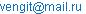(V. B. Gitis)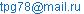(T. P. Gitis)

Veniamin B. Gitis, Tatyana P. Gitis. Improving of Procedures for Preparing of Training Set for Neural Networks. American Journal of Neural Networks and Applications. Vol. 1, No. 1, 2015, pp. 29-32. doi: 10.11648/j.ajnna.20150101.14

Abstract: In the article procedure of rough-down of information is examined for teaching of neuron networks. Shown, that exists problem of normalization of ordinals of variables in part of their internal levels. The improved chart of normalization, allowing setting ponder ability both ordinal of variable on the whole and its separate levels, is offered to application. Reverse normalization formulas over are also brought for interpretation of gravimetric coefficients of neurons.

Keywords: Neuron, Normalization, Ordinal Variable, Neural Network, Self-Organizing Maps

Contents

1. Introduction

Usually components of input vectors have different units of measure and ranges of variation. Some of the variables are represented by continuous quantity, which changes within a limited range. Other variables are discrete (for example, integer) and are measured in a uniform scale. That is, for such variables the distance between the adjacent values is known and it is constant (equal to one). This is due to the fact that these variables reflect quantitative characteristics which are obtained by the accumulation of any quantities.

There is also a group of variables obtained by qualitative variables digitizing and reflected the degree of manifestation of a quality. The ordinal scale of measurement corresponds to these variables. The known order relation between the states is the characteristic for such a scale, however, the distance between the states is not defined. Coding ordinal signs by value of one variable in the absence of a priori information about the distances between adjacent distances is difficult, because such encoding sets these distances .

2. Problem Formulation

The account of the totality of characteristics of the input signal and the perception of it as a single image information require to perform certain procedures for data preprocessing.

It is necessary to perform a scaling operation to present all the elements of the input signal the number of one type from one range.

The putting a neural network of not scaled data complicates the study of networks and leads to errors in the work, because

1.   Work of weighting coefficients of network at different scales complicates initial initialization of weights;

2.   The weighting coefficients of neurons will be very large or very small values depending on the magnitude of the dispersion, it will increase learning time and reduce the accuracy of the forecasts;

3.   The input layer neurons will be in constant saturation due to the large average value of aggregate input data and a small dispersion, or will be inhibited because of the small average sample .

It is also necessary to scale of reference output signals because usually the range of output values of neurons lies in the limited range and the neural network will not be able to answer the real data.

Since the neural networks analyze not the absolute values of input signals and their changes, you should perform a shift input data to improve the legibility of signals other than the zoom during pre-treatment data. The shift is provided in the identification of the boundaries of the range change feature and considers them as the boundaries of the input range .

Shifting and scaling operations together represent normalization input data. Numeric signals must be scaled and shifted so, that the whole range of initial values falls in the normalized range of the input signal. Original signals are normalized to the unit hypercube or hypercube with the coordinates of angles a and b on a coordinate axis corresponding to the scalable attribute, it depends on the type of neuron’s activation functions.

Purpose of work is improving the procedure for pre-processing data for learning neural network by obtaining the possibility of weight management variables of all types.

3. Main Results

The minimax conversion is used to normalize the data in a predetermined range, which is calculated by the following formula :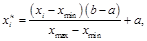(1)

where xi– normalized values;

i–the index of vector’s component of the original data (i = 1…n);

n –dimension of an input signal;

хmin, хmax– the minimum and maximum values of the normalized feature;

a, b – respectively lower and upper limits of the normalized range.

Formula (1) can be written as: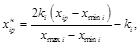(2)

if you use a range of normalization, which is symmetric around zero, when a = -b, and taking b = k, where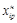– normalized p-th value of the i-th component of the vector of initial data, which will be applied to the input of the network;

xip– p-th value of the i-th component of the vector of initial data;

p – number of example in the training set (p = 1 ... P);

P –number of examples;

ki– weighting coefficient of i-th factor.

Weighting coefficient allows you to control the importance of each input factor. The baseline value is taken as k = 1,for all variables and then the output normalized range will be [1,1]. The increase of this coefficient for some factor (or reduction of the coefficient for other factors) increases the distance between the points and it allows you to emphasize the difference between objects, it is based on the value of this factor. Management of coefficients importance of factors allows ranking their role in importance for the problem.

Application of the formula (2) solves the problem of normalization and weight management of continuous variables. However, the ordinal variables must differ from continuous by the method of encoding and normalization, because it is necessary to be able tomanage not only an important factor in the whole but also the distances between the levels of ordinal variable. This will allow increasing or decreasing the importance of individual levels of criterion that is based on the characteristics of the modeled object [5,6].

It is offered to use the following formula for values modification of level ordinal variable for obtaining this possibility :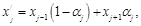(3)

where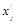– the modified value of the j-th level of ordinal variable;

xj-1and xj + 1 – values of the previous and subsequent levels of ordinal variable with respect to modifiable;

αj– weighting factor of the i-th level of ordinal variable (αÎ[0;1]);

j – level’s number of ordinal variable (j = 1 ... m);

m –a number of levels in ordinal variable.

For all levels α = 0,5 corresponds to a uniform scale normalization of ordinal variable. Reducing of αj approximates the j-th level to (j-1)-th and thus reduces its value. And also increasing of αj shifts it to the (j + 1)-th level and weight of level increases.

Taking into account the ascending order of levels of ordinal variables and based on formulas (2) and (3), formula for normalization of internal levels takes the form: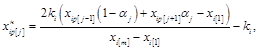(4)

whereand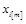– the values of the first and the last (m-th) level of ordinal variable, which correspond to the minimum and maximum values of this variable.

Normalization of the first and last level of ordinal variable is carried out according to the formula (2).

Thus, the general scheme of intermediate minimax normalization of training set has the form [8,9]: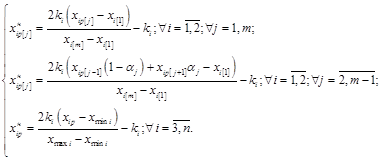(5)

The proposed processing of data will not only correctly display the input information to the neural network, but will also provide an opportunity to consider features of the modeled object.

Reverse transition from minimax conversion (interpretation) is carried out by the following formula: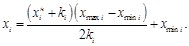(6)

Formula (6) is used for continuous output signals which are subjected to the normalization procedure according to the formula (2), and also for the boundary levels (1 and m-th) of ordinal variables.

It is necessary to calculate the current ratio of the ordinal variable α* for the interpretation of internal levels of ordinal variables, this coefficient is defined according to the relative position of a received signal level. That is, the coefficient α* shows the location of the value between the nearest (smaller and larger) normalized levels of ordinal variable. The coefficient α* can be calculated according to the formula :(7)

where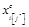– a value which is interpreted (the output signal network);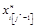and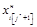– next to the valuethe lower and upper normalized values of the ordinal variable;

j*– conditional (non-integer) position in the ordinal variable.

So we have the inequality: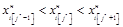Then the interpretation of signal to range of real values can be performed according to the formula:(8)

where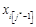and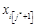- value of the ordinal variable in the real amount of data corresponding to the normalized itemsand.

A continuous quantity will be received as a result of application the formulas (7) and (8)in general.It is located in a discretescale of the ordinal variable.Such a continuous numbercan carry additional information about the obtained solution, which can be used in further analysis of the results. We should roundthe resulting number to the nearest standardized position of the ordinal variable for practical application.

4. Conclusions

1.   It is necessary to carry out pre-processing of data in order to ensure perception ofheterogeneous information by neural networks. The introduction to the procedure of preprocessing minimax conversion allows not only leading variables to a single scale, but also allows managing weight of continuous variables.

2.   The normalization method of ordinal variables must provide the ability to manage notonly an importance of factor in general, but also the distances between the levels of ordinal variable. For this formula of modification values levels of the ordinal variable are offered, which allows increasing or decreasing the importance of individual levels of criterion, it is based on the characteristics of the current task.

3.   The proposed conversion formulas of normalized values to the range of real data allow to perform the analysis of the weight values coefficients of neurons, for example, to determine the positions of the nuclei in the feature space.

References

1. Mirkes E. M. Nejrokomp'yuter: proekt standarta / E. M. Mirkes; red. V. L. Dunin-Barkovskij; RAN, SO, In-t vychisl. modelirovaniya. – M.: Nauka: Sib. predpriyatie RAN, 1999. – 190 s.
2. Zaencev I. V. Nejronnye seti: osnovnye modeli. – Voronezh: VGU, 1999. – 157 s.
3. Gorban' A. N. Nejroinformatika / A. N. Gorban', V. L. Dunin-Barkovskij, A. N. Kirdin. – Novosibirsk: Nauka. Sibirskoepredpriyatie RAN. – 1998. – 296 s.
4.   Caregorodcev V. G. Optimizaciya predobrabotki dannyh: konstanta Lipshica obuchayushchej vyborki i svojstva obuchennyh nejronnyh setej // Nejrokomp'yutery: razrabotka i primenenie.–2003.–№ 7.–S. 3-8.
5.   Gitis T. P. Analiz urovnya professional'nogo razvitiya stanochnikov s ispol'zovaniem kart Kohonena / T. P. Gitis // Sbornik trudov Mezhdunarodnoj nauchnoj konferencii «Nejrosetevye tekhnologii i ih primenenie». – Kramatorsk: DGMA. – 2012. – S. 34-38.
6. Es'kov A. L. Upravlenie professional'nym razvitiem personala predpriyatiya na osnove ego ocenki / A. L. Es'kov, T. P. Gitis // Ekonomіka ta pravo. – 2013. - №2(36). – S.87-92
7. Gitis T. P. Intellektual'nye metody upravleniya personalom predpriyatiya: monografiya / T. P. Gitis, V. B. Gitis. - Kramatorsk, DGMA, 2014. – 140 s.
8. Gitis T. P. Predvaritel'naya obrabotka dannyh dlya ocenki urovnya professional'nogo razvitiya rabochih s pomoshch'yu kart Kohonena / T. P. Gitis // Iskusstvennyj intellekt. Intellektual'nye sistemy: Materialy X mezhdunarodnoj nauchno-tekhnicheskoj konferencii. – Taganrog: Izd-vo TTI YUFU, 2009. – S. 74-76
9. Gitis T. P. Pred- i postobrabotka informacionnyh potokov samoorganizuyushchihsya kart priznakov / T. P. Gitis, V. B. Gitis // Materialy Mezhdunarodnoj nauchno-tekhnicheskoj konferencii «Iskusstvennyj intellekt. Intellektual'nye sistemy II-2013». −Doneck: IPII «Naukaіosvіta», 2013. – S. 265-267.
10. Gitis T. P. Sovershenstvovanie procedury podgotovki obuchayushchih mnozhestv dlya nejronnyh setej / T. P. Gitis, V. B. Gitis // Vіsnik Donbas'koї derzhavnoї mashinobudіvnoї akademії. – 2011. – № 2 (8E). – S. 42-46.

 Contents 1. 2. 3. 4.
Article ToolsAbstractPDF(149K)